Fast.ai Lesson 6 notes — Part 1 v3

https://course.fast.ai/

Platform.ai

This makes it easier to label image data and build simple models without coding. It is working so well because humans can see a lot of things same time which makes it plausible to see that in some part of the screen there are a lot of cat images and somewhere else there are dog images. Jeremy showed how the website is working and I think some level of introduction is good to fully understand the idea. Some of the things don’t come naturally at the beginning but after training a while it will save you a lot of time if you need to label images.

Rossmann competition

We are not going to learn how to clean the data in this tutorial. It is done for us in rossman_data_clean.ipynb If you are interested in the details, Jeremy recommended watching the machine learning course.

When someone says “time series data” often people will think about RNNs. Jeremy explained that RNN is doing better in academic datasets but in the real world, we have more information, time periods can change, etc. and that is why in practice, state of the art results are made by taking the time and creating metadata from it. For example, is it a school holiday, year, week, month, end of a week, etc. When we are using metadata, the model can learn that 15th is a payday and then people are buying more stuff.

In Fastai this is done using a function called add_datepart() If you pass in a date as a parameter, it will do all these new variables automatically.

Transforms are something which we run every time when we take a batch of data. Preprocessing is instead something we run once for all of our data before we start training. What is similar between these is that we need to use the same values for the train, test, and validation sets. When we first preprocess train data with certain values we need to use those same values for test and valid sets.

df.column_name.cat.categoriesOUTPUT:
Index(['Feb,May,Aug,Nov', 'Jan,Apr,Jul,Oct', 'Mar,Jun,Sept,Dec'], dtype='object')

df.column_name.cat.codes
OUTPUT:
280 -1
584 -1
588 1
847 -1
896 1
dtype: int8

You don’t need to run preprocesses manually. When you create TabularList object it will have procs parameter where you define preprocesses.

procs=[FillMissing, Categorify, Normalize]

Fastai assumes that you want to do classification if you pass dependent variables that are in int format. That is why you can pass label_cls parameter where you can tell that you want these to be floats and that way be handled as a regression problem.

In many cases, it is better to look at percentage differences rather than exact differences and that is why sometimes we need to use RMSPE instead of RMSE. We use this by just setting log true and then taking RMSE. Fastai is using RMSE as default for regression problems.

We can set y_range and that ways tell the model to not predict over or under some value. For example, if we are predicting prices of houses we know that price can’t be less than 0.

We set intermediate layers to go from 1,000 input activations to 500 output activations so there are 500,000 weights in that matrix. It is a lot for dataset where is only a few hundred thousand rows. This is going to overfit so we need to regularize it. Some beginners might reduce the weights but as we learned from the last lesson it is better to just regularize the model. By default, Fastai is using weight decay but for this problem (and often for other problems) we need more regularization. Regularization can be added by passing in ps and emb_drop

Dropout

The picture on the left is normal neural network and the picture on the right is the same network after applying dropout. Every arrow shows multiplications between weights and activations. A circle represents sum.

When we use dropout we throw away some percentage of the activations (NOT WEIGHTS/PARAMETERS). For each mini-batch, we throw away different activations. Amount of activations we are going to ignore is p which can be 0.5

In the picture, some of the inputs are also deleted but that isn’t common practice anymore. When we are overfitting it means that some part of our model is learned to recognize some particular image and not features as it should. When we use dropout it will assure that this can’t happen. Having too much dropout will reduce the capacity of the model.

In Fastai, ps means that we can add multiple dropouts for different layers same way we can add multiple learning rates.

We turn off the dropout (and other regularization methods) when we are testing the model. But then we have two times more parameters. In the paper, researchers suggested multiplying all weights with p. In PyTorch and many other libraries, this multiplying is done during the training so we don’t need to care about it.

emb_drop is dropout for embedding layer. We use special dropout for embedding layer because it can be a little bit higher.

BatchNorm1d

First BatchNorm takes activations. Then it takes mean and variance of those, and using those values it will normalize. Finally, (this is important) we instead of just adding bias, multiply the activations with something which is like bias. By using BatchNorm, loss decrease more smoothly and that way can be trained using higher learning rate.

Why this multiplication bias thing is working so well? Let’s say that we are again predicting movie reviews that are between 1–5. Activations in the last layer are between -1–1. We should make a new set of weights where mean and spread is increased. We can’t just move up the values because the weights are interacting very differently. With bias, we can increase the spread and now with BatchNorm, we can increase scale. Details don’t matter that much but the thing you need to know is that you want to use it. There is some other type of normalizations nowadays but BatchNorm might be the best. Jeremy told that Fastai library is using also something called WeightNorm which is developed in the last couple of months.

We create for each continues variable own BatchNorm and then run those. One thing Jeremy pointed is that we don’t calculate own mean and standard derivation for every mini-batch but rather take an exponentially weighted moving average of mean and standard derivation. We tune that by changing the momentum parameter (which isn’t the same as momentum regularizer). A smaller value will assure that mean and standard derivation doesn’t change so much and vice versa.

When to use these techniques:

• weight decay — With or without dropout depending on the problem. (Test which is working best)
• BatchNorm — Always
• dropout — With or without weight decay depending on the problem. (Test which is working best)

In general, it is often good to have a little dropout and weight decay.

Next, we are going to look at data augmentation. It is also a regularization technique. It might be the least studied regularization although there is no cost which means that you can do it and get better regularization without needing to train longer or risk to underfit.

Data augmentation

tfms = get_transforms(a_lot_of_different_parameters_to_tune)

What are the parameters?

• p_affine probability of affine transform
• p_lighting probability of lighting transform
• max_rotate how much rotate (left and right angle)
• max_zoom how much to max zoom in.
• max_warp how much warp the image.

More about these and other parameters check the docs.

One thing researcher hasn’t figure out is how to do data augmentation on other types of problems, like tabular data.

CNN

After training a CNN model we want to see what is happening there. We are going to learn what is happening by creating heatmap.

There is prebuild function for this in Fastai but Jeremy is going to show how to make it without Fastai.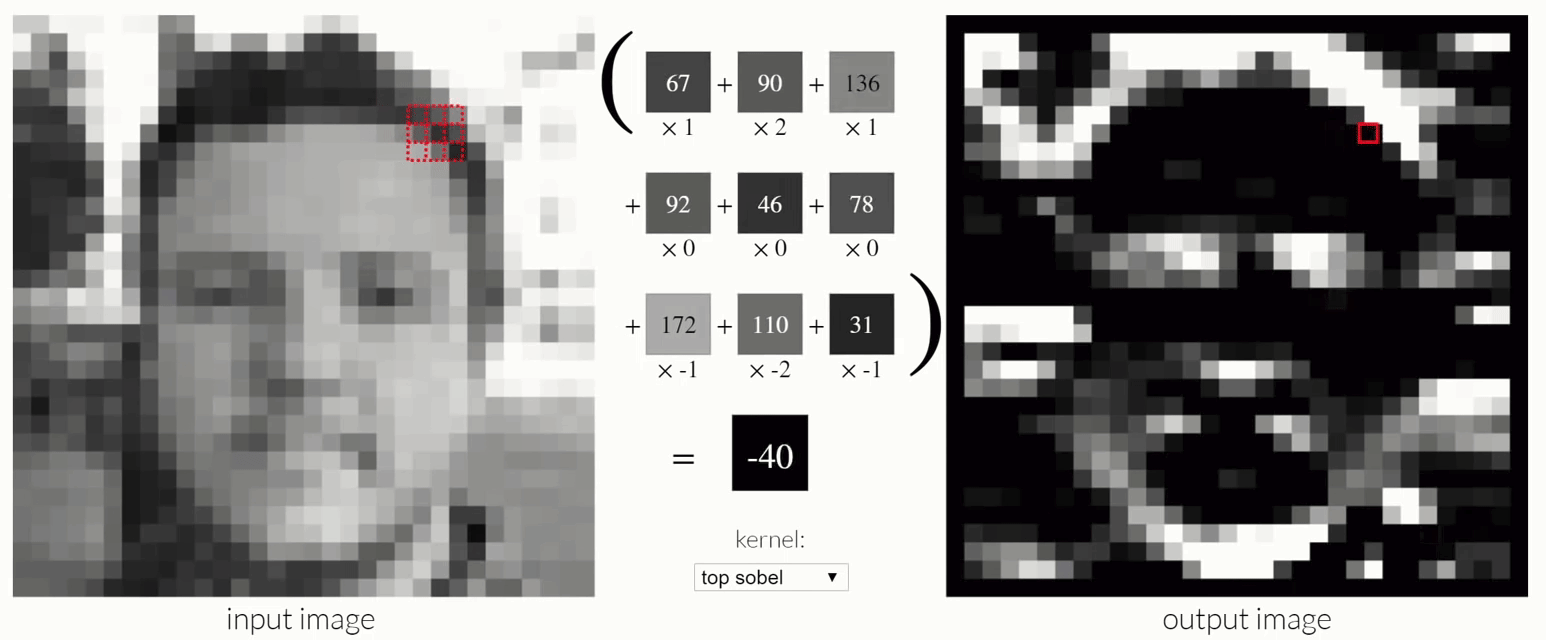http://setosa.io/ev/image-kernels/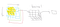This is how we do CNN if we have RGB image. Notice that although the kernel is three dimensional the output for 3x3x3 area is still one pixel.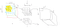We can add more kernels and combine together. 16 is a common number.

Now you are at a point where you start to understand how everything is working and that way you can use some variation of Fastai techniques. Often things in the library are designed to work generally well so you might get better results by changing some things.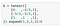We can create own kernel. Expand will make tensor 3x3x3 kernel and the first dimension is created because now we can store more than one kernel.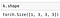First index is the number of kernels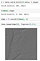Data we import conv2d need to be in batches and that is why we create one additional dimension.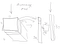Average pooling is taking the mean of every layer. Then if we want to have 37 outputs we just multiply the average pooling results with a matrix that is 37 numbers wide. Idea is that all 512 matrices are representing some feature.

When we want to create heatmap to the picture best way is to average over 512 dimensions instead of 11x11 area. That way we get 11x11 area where every pixel is average of 512 pixels. Then we can see how much that pixel activated on average.

My notes:
lesson 1 notes
lesson 2 notes
lesson 3 notes
lesson 4 notes
lesson 5 notes
lesson 6 notes
lesson 7 notes

~Lankinen